## Introduction

Prerequisites: Recursion

Recursion can sometimes be very difficult to wrap your head around because when you try to go deeper, it only keeps getting deeper. It is sometimes hard to figure out the recurrence relation, but once you do, the problem becomes simple to solve.

## Number of paths

Suppose we have a grid of N rows and M columns. How many ways are there to get from the bottom left cell to the top right cell only going upwards or rightwards?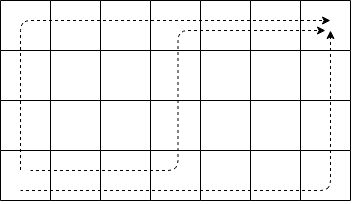We see that the only way to reach a cell, is to come from the left or from the bottom. So the number of ways to reach a cell is the number of ways to reach the cell to the left plus the number of ways to reach the cell on the bottom.

In other words, the number of ways to get to cell (x, y) is the sum of the ways to get to the cell (x-1, y) and the cell (x, y-1). However, the number of ways to reach the cell (x-1, y) is the sum of the number of ways to the cell (x-2, y) and (x-1, y-1). As we can see, we are solving a reduced version of the same problem! Thus we have a recurrence relation.

The base case is any cell on the left border or bottom border. There is only one way to reach the cell by either going only up or only going right.

### Formalization

```Let path(x,y) be the number of ways to get to the grid at x and y

Base case:
paths(1,y) = 1
paths(x,1) = 1

Recurrence:
paths(x,y) = paths(x-1,y) + paths(x,y-1)

Example:
paths(3,5)
= paths(2,5) + paths(3,4)
= paths(1,5) + paths(2,4) + paths(2,4) + paths(3,3)
= 1 + paths(1,4) + paths(2,3) + paths(1,4) + paths(2,3) + paths(2,3) + paths(3,2)
= 1 + 1 + paths(1,3) + paths(2,2) + 1 + paths(1,3) + paths(2,2) + paths(1,3) + paths(2,2) + paths(2,2) + paths(3,1)
= 1 + 1 + 1 + paths(1,2) + paths(2,1) + 1 + 1 + path(1,2) + paths(2,1) + 1 + paths(1,2) + paths(2,1) + ....
= 15
```

Example of what the grid should look like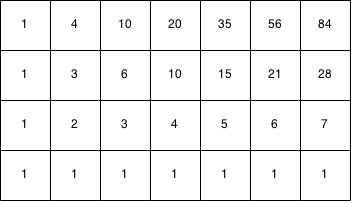### Implementation

```int paths(int n,int m){
if (n == 1 || m == 1) {
return 1;
}
return paths(n - 1, m) + paths(n, m - 1);
}
```

## Towers of Hanoi

Suppose we have a game where there are three poles and N discs where each disc is heavier than the next disc. In the initial configuration, the discs are stacked upon another on the first pole where the lighter discs are above the heavier discs. The end goal is to move all the discs to the last pole with the following conditions:

• Only one disc can be moved from one pole to another at a time.
• The discs have to be stacked such that all the lighter discs are on top of the heavier ones.

Let's try to make this problem simpler. To move N discs from the first pole the the last pole we need to move N-1 discs to the middle pole, then move the Nth disc to the last pole, and then move all N-1 discs from the middle pole back on to the last pole.

Let the starting pole be the first pole, the helper pole be the middle pole and the destination pole the third pole.To move N discs from the starting pole to the destination pole:

Step 1:

We need to move N-1 discs from the starting pole to the helper pole.Step 2:

We need to move the Nth disc from the starting pole to the destination pole.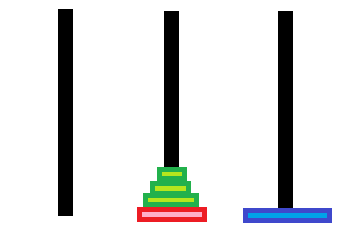Step 3:

We need to move N-1 discs from the helper pole to the destination pole.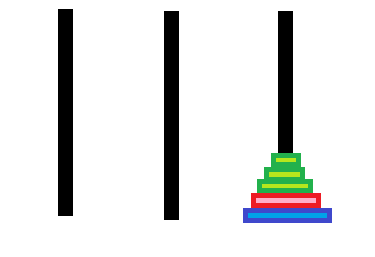We can see that Step 2 is easy, all we have to do is move one disc. But for Step 1 and Step 3, we have to move N-1 discs. How can we move N-1 discs to the middle pole?

We see that we can use the same reasoning: we need to move N-2 discs to the third pole. Then we need to move the N-1 disc to the second pole and then move N-2 discs from the third pole to the second pole. Note that the Nth disc does not matter at all in this case since we can move any disc on top of it and we can pretend as if it doesn't exist.

In this case, the starting pole is the first pole, the helper pole is the third pole and the destination pole is the middle pole.To move N-1 discs from starting pole to destination pole:

Step 1:

We need to move N-2 discs from starting pole to helper pole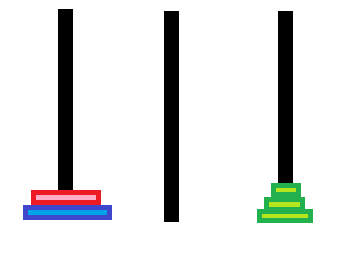Step 2:

We move the N-1th disc from the starting pole to the destination pole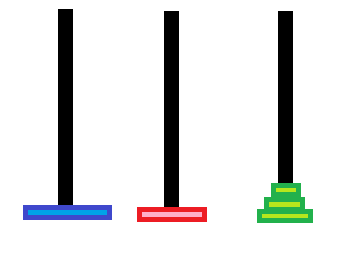Step 3:

We move N-2 discs from the helper pole to the destination pole.As we can see, the steps to move N discs are exactly the same as to move N-1 discs! The only difference is that the actual poles are different but the steps are the same relative to their roles (starting, helper and destination). We are solving a reduced version of the same problem, thus we have a recurrence.

In Step 1, when we move N-1 discs from start to helper, the new helper is the old destination and the new destination is the old helper.

In Step 3, when we move N-1 discs from the helper to the destination, the new helper is the start pole, and the new start pole is the helper

### Formalization

```Let hanoi(N, start, helper, dest) print the steps to move N discs from the start to dest

Base case:
hanoi(1, start, helper, dest):
print "Move from (start) to (dest)"

Recurrence:
hanoi(N, start, helper, dest):
hanoi(N - 1, start, dest, helper)
print "Move from start to dest"
hanoi(N - 1, helper, start, dest)

Example:
Let A, B, C be pole 1, 2, 3

hanoi(4,A,B,C)

=

hanoi(3,A,C,B)
Move from A to C
hanoi(3,B,A,C)

=

hanoi(2,A,B,C)
Move from A to B
hanoi(2,C,A,B)
Move from A to C
hanoi(2,B,A,C)
Move from B to C
hanoi(2,A,B,C)

=

hanoi(1,A,C,B)
Move from A to C
hanoi(1,B,A,C)
Move from A to B
hanoi(1,C,B,A)
Move from C to B
hanoi(1,A,C,B)
Move from A to C
hanoi(1,B,C,A)
Move from B to C
hanoi(1,A,B,C)
Move from B to C
hanoi(1,A,C,B)
Move from A to C
hanoi(1,B,A,C)

=

Move from A to B
Move from A to C
Move from B to C
Move from A to B
Move from C to A
Move from C to B
Move from A to B
Move from A to C
Move from B to A
Move from B to C
Move from A to C
Move from B to C
Move from A to B
Move from A to C
Move from B to C

```

### Implementation

```void hanoi(int N, int start, int helper, int destination) {
// Base case to move one disk.
if (N == 1) {
System.out.println("Move " + start + " to " + destination);
}
else {
// Move N-1 discs from start to helper.
hanoi(N - 1, start, destination, helper);

// Move 1 disc from start to end.
hanoi(1, start, helper, destination);

// Move N-1 discs from helper to end.
hanoi(N - 1, helper, start, destination);
}
}
hanoi(4, 1, 2, 3);
```

## Permutations

A permutation is an arrangement of the original set of elements.

For example, here we have a few permutations of A,B,C,D,E,F:

• D,E,F,C,B,A
• F,C,D,B,A,E
• B,D,A,E,F,C
• A,B,C,D,E,F

Given a string S of length N, how can we generate all permutations?

Let's assume that we have a list of permutations for the substring of S of N-1 characters. To get the permutations for a string of length N, we need to insert the Nth character in between all the positions of each permutation of N-1 characters. For example, here is how the permutation of A,B,C,D is generated.

We can manually find the permutations of A,B,C.

• A,B,C
• A,C,B
• B,A,C
• B,C,A
• C,A,B
• C,B,A

If we insert the letter D between each letter for every permutation in N-1 letters, we get:

• D,A,B,C
• A,D,B,C
• A,B,D,C
• A,B,C,D
• D,A,C,B
• A,D,C,B
• A,C,D,B
• A,C,B,D .... etc

And we can guarantee that every new permutation will be unique. (Try to prove that to yourself).

But let's look at how we can get the permutations of A,B,C. We can also get all the permutations of the string by taking the permutations of A,B and inserting C in all the positions for all substrings.

Substrings of A,B

• A, B
• B, A

Insert C for all positions for all permutations

• C,A,B
• A,C,B
• A,B,C
• C,B,A
• B,C,A
• C,B,A

For simplicity, S[i..j] will mean the substring from i inclusive to j exclusive. For example, if S = "abcd", S = 'a' and S[1..4] = "bcd".

We see that we are solving the same reduced problem, thus we have a recurrence relation. To get the permutations of a string N, we take the string[0..N-1] and we insert the Nth character at every position for each permutation of the N-1 substring. The base case is an empty string. Permutation of an empty string is an empty list.

```Let permute(S) be a list of permutations for the string S

Let insertAll(ch, stringArr) be a function that inserts the character ch in every position in every string stringArr.

Example:
insertAll('c', ['ab','ba']) = ['cab', 'acb', 'bac', 'cba', 'bca', 'bac']

Base case:
permute("") = [""]

Recurrence:
Let N be the length of string S
permute(S) = insertAll(S, permute(S[1..N]) )

Example:
permute('ABC')
= insertAll('A', permute('BC')
= insertAll('A', insertAll('B', permute('C'))
= insertAll('A', insertAll('B', insertAll('C', permute(''))))
= insertAll('A', insertAll('B', insertAll('C', [''])))
= insertAll('A', insertAll('B', ['C']))
= insertAll('A', ['BC', 'CB'])
= ['ABC', 'BAC', 'BCA', 'ACB', 'CAB', 'CBA']
```

### Implementation

```Vector<String> insertAll(char ch, Vector<String> strArr) {
Vector<String> vec = new Vector<String>();
for (int i = 0; i < strArr.size(); i++) {
String str = strArr.get(i);
for (int j = 0; j <= str.length(); j++) {
vec.add(str.substring(0, j) + ch + str.substring(j, str.length()));
}
}
return vec;
}

Vector<String> permutation(String s) {
int n = s.length();
// Base case of one empty string.
if (n == 1) {
Vector<String> vec = new Vector<String>();
return vec;
}
// Recursive relation.
return insertAll(s.charAt(0), permutation(s.substring(1)));
}

// Example usage:
permutation("abc");
```

## Exercises

1. Given a string S, write a recursive function to generate all its non-empty substrings.
2. Write a solution for hanoi towers but with the restriction that discs can only be moved from adjacent poles. (You can move a disc from A to B but not A to C because they are not adjacent).
3. Write the formalization and code for a recursive insertAll(ch, stringArr).